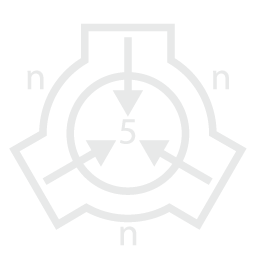nn5n Foundation
Branch of SCP Foundation
nn5n: scp-1941 Lunar Von Neumann Catastrophe
EuclidSCP-1941 Lunar Von Neumann CatastropheRate: 81SCP-1941SCP-1941 phenomenon (photo provided by LRO). The black area resembling a network is the result of ongoing construction.Detail of an individual node and its excavations. The network is self-similar: individual nodes resemble the network at large. Depicted here is the equivalent of a single node in the preceding photograph. This image is at the furthest limit of the LRO's resolving power (crater is approximately 50m wide).

Item #: SCP-1941

Object Class: Euclid

Special Containment Procedures: Due to its location, SCP-1941 is uncontainable for the time being. Of highest priority is the development of advanced lunar reconnaissance orbiters to continue to track its progress, as well as a lunar retrieval mission in order to procure a sample for study. For the moment, the phenomenon is not visible from the Earth, making a cover story unnecessary.1

Description: SCP-1941 is a manifestation that was first discovered on May 28, 2000 when attention was drawn to what had been assumed to be a small comet impacting 500km south-east of Jackson crater in the Northern hemisphere of the far side of the moon. Since its discovery, evidence of rapid industrial activity and exponential growth have emerged.

The currently deployed Lunar Reconnaissance Orbiter's (LRO) resolving power is insufficient to determine exactly what is responsible. However, anomalously high temperatures and spectroscopy results suggest that strip-mining, refining,  and fabrication are taking place. In addition, neutrino activity consistent with controlled nuclear fusion has been detected.

It has been conjectured that a technologically sophisticated alien package impacted the moon and is responsible for SCP-1941. Such a package (known as a Von Neumann probe) would be able to make use of available raw materials in its environment in order to replicate itself. When enough copies had been produced, it could then turn its attention to some longer term goal.

In the decade since it was discovered, the area the phenomenon encompasses has grown by a factor of 3.5, giving it an estimated doubling rate of 7 years. It currently occupies an area of 250,000 km2 (6% of the moon's surface, or roughly the area of the United Kingdom). If it continues to spread at the same rate, it will begin to be visible from Earth in August of 2023. The entire moon's surface would be covered by 2040.

Addendum: Beginning in June of 2002, transmissions from the site were detected. They consisted of a bootstrap linguistic program that established a small mathematical vocabulary, followed by a particular number and a request for its prime factors. It is currently unknown what the consequences are of either transmitting or failing to transmit these factors. Unfortunately, the number in question is intractably large and not amenable to factorization by any conventional means.

It is not known if the difficulty posed by such a mathematical problem is meant as an intelligence test, or if the civilization responsible for SCP-1941 has drastically over-estimated humanity's abilities. The possibility exists that this is meant to measure a civilization's sophistication and to judge the level of resistance they could bring to bear were SCP-1941's intentions in fact hostile.

What follows is the expression for the number in question:

$\large 2^{2^{79}} + 3^{2^{83}} + 5^{2^{89}} + 7^{2^{97}}$

Recent Developments: In March of 2014, a secondary message was detected in what was thought to be noise in the primary channel. Using the established mathematical vocabulary, the secondary message appears to suggest that the transmission of the aforementioned prime factors would signal the lunar phenomenon to halt. It has been conjectured that SCP-1941 was designed to be shutdown by a neighboring civilization of sufficient sophistication should they find it's activities undesirable.

Given this development, interest has been renewed in attempting to factor the mathematical expression in the primary message. Investigations into leveraging the processing power of SCP-155 were made (SCP-155 being a computer capable of an asymptotic number of computations in finite amounts of time), as well as investigations into the attendant risks of doing so (see documentation for SCP-155).

Estimates have been made on the amount of energy that would be released by SCP-155 during this attempt, and whether or not provisions should be made to relocate it off the Earth should it prove necessary to safeguard against a PK-class event, or the sterilization of all life on the planet.

The lower-bound placed on the amount of energy released is 4.2 x 1018 Joules,  deemed acceptable (roughly equivalent to a gigaton nuclear explosion). The upper-bound, however, has been placed at 3.1 x 1044 Joules, or roughly the amount of energy released by the average supernova. Research is currently underway to refine these bounds.

Mathematical Supplement:
The secondary message is as follows:

(1)
\begin{align} \large f(): f(n) =  {p_1}^{a_1}{p_2}^{a_2} \dotsb {p_k}^{a_k} , \forall p  \neg \exists a,b :  a>1,  b>1, p=ab \end{align}
(2)
\begin{align} \large \Omega = 2^{2^{79}} + 3^{2^{83}} + 5^{2^{89}} + 7^{2^{97}} \end{align}
(3)
\begin{align} \large f(n) \Rightarrow \left\{ \begin{array}{rl} n=∅ &\mbox{-> e} \\ n=Ω &\mbox{-> 0} \end{array} \right. \end{align}

Which has been interpreted to mean the following:

(1):   Definition of the function f() which yields the prime factors of an integer.
(2):   Definition of Ω, the intractably large number.
(3):   The condition, where the prime factors of the empty set f(∅) yields the base of the natural logarithm, e (interpreted to mean continued exponential growth) while the prime factors of the intractable number f(Ω) yields 0, (interpreted to mean the cessation of growth).

Upper and Lower bounds:
The lower bound is estimated using the expectation that, on average, as the number Ω approaches infinity,  Ω will have $\ln\ln$ Ω number of prime factors. The upper bound is estimated using the assumption that the number Ω represents the pathological case and is itself prime.

page revision: 107, last edited: 03 Mar 2016 22:27
Unless otherwise stated, the content of this page is licensed under Creative Commons Attribution-ShareAlike 3.0 License